# Potential of a mass distribution

An expression of the form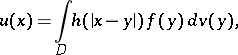(*)

whereis a bounded domain in a Euclidean space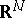,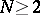, bounded by a closed Lyapunov surface(a curve for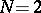, cf. Lyapunov surfaces and curves),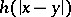is the fundamental solution of the Laplace operator:where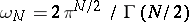is the area of the unit sphere in,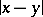is the distance between the pointsand, and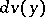is the volume element in.

If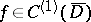, then the potential is defined for all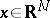and. In the complementary domain, the functionthen has derivatives of all orders and satisfies the Laplace equation: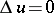, that is, is a harmonic function; forthis function is regular at infinity,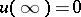. Inthe potentialbelongs to the class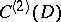and satisfies the Poisson equation:.

These properties can be generalized in various ways. For example, if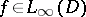, then,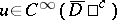,in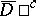,has generalized second derivatives in, and the Poisson equationis satisfied almost-everywhere in. Properties of potentials of an arbitrary Radon measureconcentrated on an-dimensional domainhave also been studied: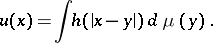Here againandin,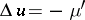almost-everywhere in, where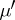is the derivative ofwith respect to Lebesgue measure in. In definition (*) the fundamental solution of the Laplace operator may be replaced by an arbitrary Levi function (see ) for a general second-order elliptic operatorwith variable coefficients of class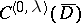; then the properties listed above still hold with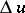replaced by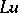(see ).

Potentials of mass distributions are applied in the solution of boundary value problems for elliptic partial differential equations (see ).

For the solution of boundary value problems for parabolic partial differential equations the concept of a heat potential of the formis used, where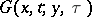is a fundamental solution of the heat equation in: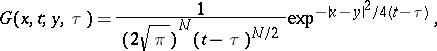and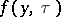is the density. The functionand its generalizations to the case of an arbitrary second-order parabolic partial differential equation have properties similar to those given above for(see ).